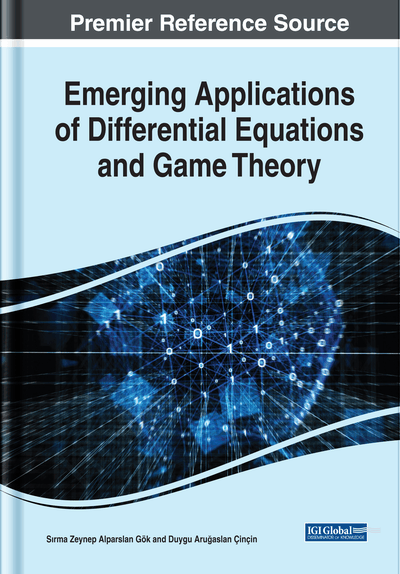On the Orthogonality of the q-Derivatives of the Discrete q-Hermite I Polynomials

Sakina Alwhishi (El Mergib University, Libya), Rezan Sevinik Adıgüzel (Atılım University, Turkey) and Mehmet Turan (Atilim University, Turkey)
DOI: 10.4018/978-1-7998-0134-4.ch007
Available
\$37.50
No Current Special Offers

Abstract

Discrete q-Hermite I polynomials are a member of the q-polynomials of the Hahn class. They are the polynomial solutions of a second order difference equation of hypergeometric type. These polynomials are one of the q-analogous of the Hermite polynomials. It is well known that the q-Hermite I polynomials approach the Hermite polynomials as q tends to 1. In this chapter, the orthogonality property of the discrete q-Hermite I polynomials is considered. Moreover, the orthogonality relation for the k-th order q-derivatives of the discrete q-Hermite I polynomials is obtained. Finally, it is shown that, under a suitable transformation, these relations give the corresponding relations for the Hermite polynomials in the limiting case as q goes to 1.
Chapter Preview
Top

Introduction

The so-called q.polynomials have great applications in several problems on theoretical and mathematical physics, for example, in the continued fractions, Eulerian series (Fine, 1988), algebras and quantum groups (Koornwinder, 1995, 1994; Vilenkin & Klimyk, 1998), discrete mathematics, algebraic combinatorics (coding theory, design theory, various theories of group representation) (Bannai, 1990), q.Schrödinger equation and q.harmonic oscillators (Askey et al., 1993; Askey & Suslov, 1993; Atakishiyev & Suslov, 1991; Berg & Ismail, 1994; Borzov & Damaskinsky, 2003; Macfarlane, 1989).

Discrete q.Hermite I polynomials form an important class of q.polynomials in the Hahn sense. They satisfy the following q.difference equation of hypergeometric type:

(1) where λ.is a constant which has a particular form depending on the parameter q. and Dq. is the q.Jackson derivative (Koekoek et al., 2010; Nikiforov et al., 1991; Nikiforov & Uvarov, 1986, 1988).

In fact, discrete q.Hermite polynomials are the q.analogous of the Hermite polynomials which are one of the important orthogonal family of the classical orthogonal polynomials (Koekoek et al., 2010; Nikiforov et al., 1991; Nikiforov & Uvarov, 1986, 1988; Szegö, 1939) that have enormous applications in mathematics and physics. They satisfy the following differential equation of hypergeometric type

(2) where.The Hermite polynomials and their q.analogues can be obtained by using an appropriate limit relation from the other orthogonal polynomials (Andrews et al., 1999; Koekoek et al., 2010).

The classical orthogonal polynomials and their q.analogues have several properties. For example the differential and the difference equations that they satisfy have special forms, which are studied by Bochner in 1929 (Bochner, 1929) and by Routh in 1885 (Routh, 1885). Rodrigues formula is another property that provides a representation for the classical polynomials derived by Tricomi (Tricomi, 1955) and (Cryer, 1970). The three terms recurrence relation (TRRR) is also a way to construct classical orthogonal polynomials considered by Chihara (Chihara, 1978) and Szegö (Szegö, 1939). Moreover, the generating function is another option to define these polynomials. Using generating function, such polynomials can be obtained in a series form which is first studied by Abraham and Moivre in 1730 (Koekoek et al., 2010).

Complete Chapter List

Search this Book:
Reset